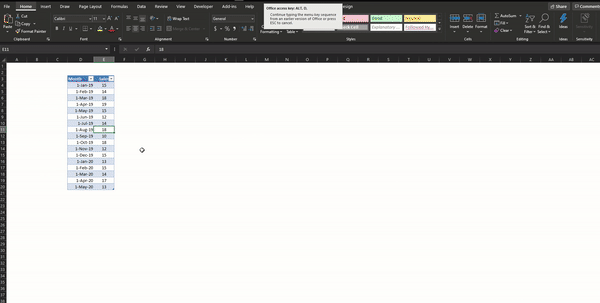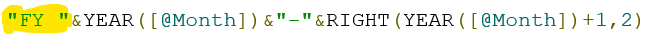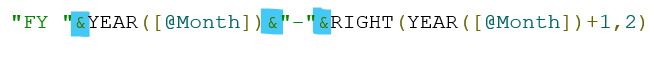Search

# Calculating Financial Year & QuarterApart from the calendar year (Jan to Dec), analysts require comparing results based on the financial year. In India, the financial year is April to March. There is no direct formula available in Excel to identify the financial year and the subsequent quarter. This article explains the common logic, which can be applied elsewhere.

Sample Data:## Summarizing it by the Calendar Year & Quarter

The pivot table is the simplest way of summarizing a table in Excel.

Select the table > Insert > Pivot TableAlternatively, we can use the keyboard shortcut Alt, D, P, & F (do not hold all the keys together)

By default, when we put the Month column under rows, Pivot group the field into the calendar year and quarter:Pivot helps in grouping data for date fieldsFor this exercise, we like to include another category of the financial year (April to March). For example, Jan 2019 is in FY 2018-19, May 2019 & Feb 2020 is in FY 2019-20.Experience says that if we explain the problem statement into simple words, it becomes an easy task to write the solution. We can write our scenario as:

If the month of the year is between 4 & 12, then it falls in the current calendar year, else in the previous calendar year (for months 1,2, & 3).

It requires the usage of IF function with the combination of MONTH, YEAR functions. We use text function RIGHT and concatenate using & for formatting the output in the desired format.

Following is the IF statement:

``` =IF(MONTH([@Month])>3,"FY "&YEAR([@Month])&"-"&RIGHT(YEAR([@Month])+1,2),"FY "&YEAR([@Month])-1&"-"&RIGHT(YEAR([@Month]),2))
```

Where [@Month] is the column name of the date column.

Let us break it down### Logical Question:

MONTH function returns the month number from a date. For example, January is 1; May is 5; October is 10.

Since our threshold for the financial year end is March (3), the IF statement is checking the month value of the date in the cell.

If in case the financial year end is other than March, then we can adjust the logical question accordingly. For example, if the threshold is May, then change 3 to 5. The rest of the formula remains unchanged.

### Output, if TRUE

The IF statement applies the following formula if the month output of the date column is between 4 & 12The structure of the financial year category is:

1. Text: “FY”

2. Start Calendar Year

3. Joining Text character: “-“

4. End Calendar Year

Part 1

To begin, we add the character FY including space:#### Part 2

The YEAR function extracts the calendar year from a date. For example, the YEAR function on 12-Jan-2019 returns the value of 2019.

For a date 31-May-2020, the start year of the financial year is 2020. To achieve this, we use the YEAR function to extract Start Calendar Year using YEAR function:#### Part 3

We add the character “-“ into the string#### Part 4

The last part of the group contains the end calendar year. For the financial year starting in 2020, the end calendar year is March of 2021. So as the first step, we add 1 to the current calendar year:And as we need only the last two characters of the end calendar year, we use the RIGHT function and extract the last two characters from the expression above:So, the right two characters of 2021 are 21.

Each part is joined by the concatenate character “&”.### Output, if FALSE

The IF statement runs this segment when the calendar month is between 1 & 3.In our scenario, months of January, February, and March falls in the previous calendar year. For example, 20-Feb-2020 comes under FY 2019-20.

Hence, we have made the following changes in the:

1. Start Calendar Year: Reduce the calendar year by 1. If the calendar year is 2020, then the output is 2019.2. End Calendar Year: Remains unchanged from the calendar yearExcel treats March as the 3rd month of the year, April as the 4th, and December as the 12th. For the financial year quarter, we want Excel to shift this by three months. April should be the 1st month, December should be the 9th month, and March should be the 12th month of the year. Hence, for the financial year Apr-Mar, dates between Apr-Jun falls in Q1, and dates between Jan-Mar falls in Q4.To achieve this, we implement the following steps:

1. Go back 3 months from the current date/month

2. Get the month number of the shifted date

3. Group all the months in a quarter

4. Get the quarter number of the financial year

5. Format it as a quarter

We use the following formula for achieving the result:

``` ="Q"&CEILING.MATH(MONTH(EOMONTH([@Month],-3)),3)/3
```

Where [@Month] is the column name of the date column.

The following table explains the step-by-step calculation### Step 1: EOMONTH

It Returns the last day of the month that is the indicated number of months before or after start_date. It has two arguments: Start_Date & MonthsThe formula EOMONTH([@Month],-3) helps in shifting the months by 3.

### Step 2: MONTH

Returns the month number of the date

### Step 3: CEILING.MATH

It is the trickiest part of the calculation.

CEILING.MATH function rounds up a number to the nearest integer or the nearest multiple of the number defined, also known as significance. If the value of significance is 3, then

CEILING.MATH function rounds up the number to the nearest multiple of 3 (3, 6, 9, 12,…). For example, 1 becomes 3, 4 becomes 6.

The following table shows a sample of the output of the CEILING.MATH function with significance value of 1,2 & 3.For our purpose, we group the months at the multiple of 3.

### Step 4: Grouping

The idea is to group all the twelve months in a year into four groups. For this, we divide it by 3 (12/3 = 4)

### Step 5: Formatting

Concatenate the output of the previous step with Q to format the quarter number as Q1, Q2, Q3 & Q4.

## Usecase

Apart from Excel, we can use this formula is applicable wherever we want to include a calculated column or a calculation in a table. We can write the same expression in DAX (Power BI & Power Pivot), Power Apps, or wherever the If Then Else works.

@imVivRan